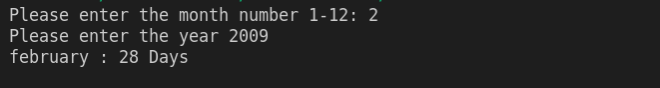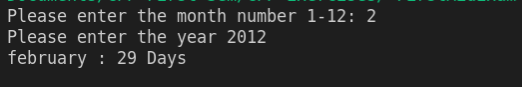Write a c++ program that takes input from the user to enter two values the month number (an integer in the range of one to twelve) and the year (a four-digit like 2010, 1000, 2015 ) The program should then display the number of days in that month.

1. You should also see if a year is a leap then display the month days accordingly.

Rules for checking whether the year is leap is not.

• if the year is a century year like 5000, 2000, 4000, 5200 then it must be divisible by 400 to be a leap year. For instance, the year 2000 is a leap year but 2100 is not, because it is not divisible by 400.
• if it is not a century year, then it should be fully divisible by 4 to be a leap year. for example, the years 2012 or 2020 are divisible by 4 so each of them is a leap year whereas 2009 or 2018 are not leap years because they are not fully divisible by 4.

You have to make sure that a valid value should be given for the month number (Between the range of 1 to 12 only)

#include <iostream>
using namespace std;

int main()
{
int months;
int years;
cout<<"Please enter the month number 1-12: ";
cin>>months;
cin>>years;

if (months <= 1 || months <= 12)
{
if (months == 1)
{
cout<<"January : 31 Days ";
}
else if (months == 2)
{
if (years % 100 == 0)
{
if (years % 400 == 0)
{
// cout<<"Leap Year\n";
cout<<"february : 29 Days ";
}
else
{
// cout<<"Not a leap year\n";
cout<<"february : 28 Days ";
}
}
if (years %100 != 0)
{
if (years % 4 == 0)
{
// cout<<"Leap Year\n";
cout<<"february : 29 Days ";
}
else
{
// cout<<"Not a leap year\n";
cout<<"february : 28 Days ";
}
}
}
else if (months == 3)
{
cout<<"March : 31 Days ";
}
else if (months == 4)
{
cout<<"April : 30 Days ";
}
else if (months == 5)
{
cout<<"May : 31 Days ";
}
else if (months == 6)
{
cout<<"June : 30 Days ";
}
else if (months == 7)
{
cout<<"July : 31 Days ";
}
else if (months == 8)
{
cout<<"August : 31 Days ";
}
else if (months == 9)
{
cout<<"September : 30 Days ";
}
else if (months == 10)
{
cout<<"Octumber : 31 Days ";
}
else if (months == 11)
{
cout<<"November : 30 Days ";
}
else if (months == 12)
{
cout<<"December : 31 Days ";
}

}
else
{c++ programming exercise with solutionc++ programming exercise with solution